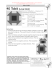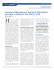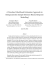# ECONOMETRICS LECTURE: HECKMAN’s SAMPLE SELECTION MODEL

## Transcription

ECONOMETRICS LECTURE: HECKMAN’s SAMPLE SELECTION MODEL
```ECONOMETRICS LECTURE: HECKMAN’s SAMPLE SELECTION MODEL
Heckman J (1979) Sample selection bias as a specification error, Econometrica,
47, pp. 153-61. Note: Heckman got the Nobel prize for this paper.
The model was developed within the context of a wage equation:
THE WAGE EQUATION
Wi = βXi + εi
(1)
where Wi is the wage, Xi observed variables relating to the i’th person’s
productivity and εi is an error term. W is observed only for workers, i.e. only
people in work receive a wage.
SAMPLE SELECTION (i.e. being in the labour force so W is observed)
There is a second equation relating to employment:
E*i
= Z iγ
+
ui
(2)
E*i = Wi – E'i is the difference between the wage and the reservation wage E'i.
The reservation wage is the minimum wage at which the ith individual is
prepared to work. If the wage is below that they choose not to work. We observe
only an indicator variable for employment defined as E=1 if E*i>0 and E=0
otherwise.
ASSUMPTIONS
The Heckman model also uses the following assumptions:
(ε,u) ~ N(0,0,σ2ε, σ2u,ρεu)
(3)
That is both error terms are normally distributed with mean 0, variances as
indicated and the error terms are correlated where ρεu indicates the correlation
coefficient.
(ε,u) is independent of X and Z
(4)
The error terms are independent of both sets of explanatory variables.
Var(u) = σ2u = 1
(5)
This is not so much an assumption as a simplification it normalises the
variance of the error term in what will be a probit regression.
THE SAMPLE SELECTION PROBLEM
Take the expected value of (1) conditional upon the individual working and the
values of X:
E(Wi | Ei=1,Xi) = E(Wi | Xi Zi ui)
(the right hand side comes from (2)
Wi = βXi + εi
(1)
E(Wi | Ei=1,Xi) = E(Wi | Xi Zi ui) =
βXi + E(εi| Xi Zi ui)
(6)
This comes from recognising that the expected value of X given X is simply X
(and the assumption that Xi is independent of the two error terms). E(X|X)=X
The final term in (6) {E(εi| Xi Zi ui) } can be simplified by noting that
selection into employment depends just on Zi and ui not upon Xi. Specifically
E(Wi | Ei=1,Xi) =
βXi + (εi| Ei =1) = βXi + (εi| ui > -Ziγ)
This is from equation (2); Ei=1 iff E*i > 0 i.e. if
Zi γ
Ziγ
(7)
+
ui > 0, i.e. if ui > -
The key problem is that in regressing wages on characteristics for those in
employment we are not observing the equation for the population as a whole.
Those in employment will tend to have higher wages than those not in the labour
force would have (that is why they are not in the labour force). Hence the
results will tend to be biased (sample selection bias) and e.g. we are likely
to get biased results when estimating say the returns to education. For example
two groups of people (i) industrious; (ii) lazy. Industrious people get higher
wages and have jobs, lazy people do not. In effect we are doing the regression
in this simplified example on the industrious part of the labour force. The
returns to education will be estimated on them alone not the whole of the
population (which includes the lazy people).
In terms of (7) the problem comes from (εi| ui > -Ziγ). The error term u is
restricted to be above a certain value, i.e. it is bounded from below. Those
individuals who do not satisfy this are excluded from the regression. OK, but
this becomes a problem because of the assumption in (3) that the error terms
are correlated where ρεu indicates the correlation coefficient. Hence a lower
bound on u suggests it too is restricted.
E(Wi | Ei=1,Xi) =
βXi + (εi| Ei =1) = βXi + (εi| ui > -Ziγ)
(7)
HECKMAN’s METHODOLOGY
Heckman’s first insight in his 1979 Econometrica paper was that this is can be
approached as an omitted variables problem (εi| ui > -Ziγ) is the ‘omitted
variable’ in (7). An estimate of the omitted variable would solve this problem
and hence solve the problem of sample selection bias. Specifically we can model
the omitted variable by:
E[(εi| ui > - Ziγ)] = ρεuσε λi(-Ziγ) = βλ λi(-Ziγ)
(8)
where λi(-Ziγ) is ‘just’ the inverse Mill’s ratio evaluated at the indicated
value and βλ is and unknown parameter (=ρεuσε)
THE INVERSE MILL’s RATIO
Many of the analyses stop there. Lets see if we can go a little further and
look at the inverse Mill’s ratio. Named after John P. Mills, it is the ratio of
the probability density function over the cumulative distribution function of a
distribution. Use of the inverse Mills ratio is often motivated by the
following property of the truncated normal distribution. If x is a random
variable distributed normally with mean μ and variance σ2, then it is possible
to show that
E(x|x>α) = μ + σ[{φ((α-μ)/σ)}/{1-Φ((α-μ)/σ)}]
(9)
where α is a constant, φ denotes the standard normal density function, and Φ
denotes the standard normal cumulative distribution function. The term in red
denotes the Inverse Mill’s ratio. Compare (9) with (8).
E[(εi| ui > - Ziγ)] = ρεuσε λi(-Ziγ) = βλ λi(-Ziγ)
(8)
•
x equates to u; hence μ, the mean of u (previously x) = 0 Also σ2 is the
variance of of u (previously x) and by (5) has been standardized to equal 1.
•
α equates to - Ziγ
Hence:
E(ui | ui > - Ziγ) =
[{φ(- Ziγ)}/{1-Φ(- Ziγ )}]
However, but we want E[(εi| ui > - Ziγ)]
(10)
not E(ui | ui > - Ziγ).
Now ρεu = σεu/(σε σu); hence ρεuσε σu= σεu; σu= 1 by definition; hence ρεuσε = σεu We
have found the expected value of ui to find the expected value of εi we must
multiply by this covariance i.e. by σεu or alternatively by ρεuσε. This gives us
E[(εi| ui > - Ziγ)] = ρεuσε. [{φ(- Ziγ)}/{1-Φ(- Ziγ )}]
(11)
Compare with: E[(εi| ui > - Ziγ)] = ρεuσε λi(-Ziγ) = βλ λi(-Ziγ)
(8).
The two are the same where λi(-Ziγ)= [{φ(- Ziγ)}/{1-Φ(- Ziγ )}]
USE IN STATA
What follows below is a special application of Heckman’s sample selection
model. That is the second stage equation is also probit. To use the standard
Heckman model where the second stage estimation involves a continuous variable
the following type of command should be used:
heckman wage educ age, select(married children educ age)
i.e. heckman rather than heckprob as we now use:
STATA COMMAND
heckprob intbankr lgnipc male age agesq rlaw estonia village town unemp selfemp
if missy==1, select(marrd educ2 lgnipc age agesq village town unemp manual
fphoneacd)
intbankr lgnipc male age agesq rlaw estonia village town unemp selfemp:
specification of variables in internet banking equation (lgnipc=log GNI per
capita; educ2 =education; marrd=married, agesq =age2; unemp=unemployed)
select(marrd educ2 lgnipc age agesq village town unemp manual fphoneacd)
specification of variables in sample selection equation (fphoneacd=quality of
fixed phone access)
Probit model with sample selection
Log pseudolikelihood = -16461.32
Number of obs
Censored obs
Uncensored obs
=
=
=
23446
14706
8740
Wald chi2(10)
Prob > chi2
=
=
1066.68
0.0000
-------------------------------------------------------------------------------------------------|
Coef.
Std. Err.
z
P>|z|
[95%
Conf. Interval]
-------------+----------------------------------------------------------------------------------intbankr
|
lgnipc |
-.1043315
.0599919
-1.74
0.082
-.2219134
.0132505
male |
.1230764
.0270944
4.54
0.000
.0699723
.1761805
age |
.0364993
.0059936
6.09
0.000
.0247522
.0482465
agesq |
-.0332365
.0072216
-4.60
0.000
-.0473905
-.0190825
rlaw |
.4961302
.0242105
20.49
0.000
.4486785
.5435819
estonia |
1.621941
.0761046
21.31
0.000
1.472779
1.771103
village |
.0422248
.0356796
1.18
0.237
-.027706
.1121556
town |
.0603227
.0332633
1.81
0.070
-.0048722
.1255175
unemp |
-.0036408
.0693268
-0.05
0.958
-.1395189
.1322372
selfemp |
.2013792
.0462062
4.36
0.000
.1108166
.2919418
_cons |
-3.207285
.2232697
-14.37
0.000
-3.644886
-2.769685
-------------+------------------------------------------------------------------------------------select
|
marrd |
.1168095
.0209772
5.57
0.000
.0756949
.1579241
educ2 |
.678366
.0148053
45.82
0.000
.6493482
.7073838
lgnipc |
.6928837
.0251465
27.55
0.000
.6435975
.7421699
age |
.0294313
.003864
7.62
0.000
.021858
.0370047
agesq |
-.0661635
.0041628
-15.89
0.000
-.0743223
-.0580046
village |
-.2005996
.024718
-8.12
0.000
-.249046
-.1521532
town |
-.0914685
.0243485
-3.76
0.000
-.1391906
-.0437464
unemp |
-.6330489
.0393924
-16.07
0.000
-.7102567
-.5558412
manual |
-.3387754
.0240658
-14.08
0.000
-.3859435
-.2916074
fphoneacd |
-.3426305
.0343699
-9.97
0.000
-.4099943
-.2752668
_cons |
-4.257136
.1210887
-35.16
0.000
-4.494465
-4.019806
-------------+----------------------------------------------------------------------------------/athrho | -.4907283
.0492128
-9.97
0.000
-.5871836
-.394273
-------------+---------------------------------------------------------------rho | -.4547943
.0390337
-.527867
-.3750381
-----------------------------------------------------------------------------Wald test of indep. eqns. (rho = 0): chi2(1) =
99.43
Prob > chi2 = 0.0000
-----------------------------------------------------------------------------rho = estimate of ρεu indicates the correlation coefficient between error terms as in
equation (3). They are negatively correlated which in the little analysis I have seen
seems quite common; the Wald test indicates the correlation is very significant. Hence we
should use Heckman’s technique.
Lets compare the sample selection equation with an ordinary probit estimation
probit useint marrd educ2 lgnipc age agesq village town unemp manual fphoneacd
if missy==1, robust
Probit regression
Number of obs
Wald chi2(10)
Prob > chi2
Pseudo R2
Log pseudolikelihood = -11223.734
=
=
=
=
23446
6089.29
0.0000
0.2751
-------------------------------------------------------------------------------------------------useint |
Coef.
Std. Err.
z
P>|z|
[95%
Conf. Interval]
-------------+----------------------------------------------------------------------------------marrd |
.1000444
.0212827
4.70
0.000
.058331
.1417578
educ2 |
.6817908
.0147544
46.21
0.000
.6528726
.7107089
lgnipc|
.6925599
.0251583
27.53
0.000
.6432505
.7418693
age |
.03065
.0038641
7.93
0.000
.0230765
.0382236
agesq |
-.0674414
.0041688
-16.18
0.000
-.0756122
-.0592706
village |
-.2000183
.0247413
-8.08
0.000
-.2485104
-.1515263
town |
-.0903838
.0243895
-3.71
0.000
-.1381863
-.0425813
unemp |
-.6339594
.0394163
-16.08
0.000
-.7112139
-.5567049
manual |
-.3300255
.0246335
-13.40
0.000
-.3783062
-.2817448
fphoneacd |
-.3346584
.0350862
-9.54
0.000
-.4034261
-.2658907
_cons |
-4.28472
.1210864
-35.39
0.000
-4.522045
-4.047396
--------------------------------------------------------------------------------------------------.Taking first three lines of sample selection model we get:
marrd |
.1168095
.0209772
5.57
0.000
educ2 |
.678366
.0148053
45.82
0.000
lgnipc |
.6928837
.0251465
27.55
0.000
and probit
marrd |
educ2 |
lgnipc |
.1000444
.6817908
.6925599
.0212827
.0147544
.0251583
4.70
46.21
27.53
0.000
0.000
0.000
.0756949
.6493482
.6435975
.1579241
.7073838
.7421699
.058331
.6528726
.6432505
.1417578
.7107089
.7418693
The two are very similar. I believe the two are not identical because STATA
estimates both equations together in a maximum likelihood process.
NOTE:
select(...) specifies the variables and options for the selection equation.
is an integral part of specifying a selection model and is required. The
It
selection equation should contain at least one variable that is not in the
outcome equation.(This is true in general not just for STATA)
If the dependent variable for the selection equation is specified, it should be
coded as 0 or 1, 0 indicating an observation not selected and 1 indicating a
selected observation. If it is not specified [as above], observations for which
(in this case Internet banking) is not missing are assumed selected, and those
for which it is missing are assumed not selected. NOTE our dependent variable
is Internet banking amongst those who have access to the Internet, i.e. it is
HECKMAN ‘BY HAND’
Do probit first stage regression on full sample
probit useint marrd educ2 lgnipc age agesq village town unemp manual fphoneacd
Probit regression
Log likelihood = -12320.022
Number of obs
LR chi2(10)
Prob > chi2
Pseudo R2
=
=
=
=
24713
8194.75
0.0000
0.2496
-----------------------------------------------------------------------------useint |
Coef.
Std. Err.
z
P>|z|
[95% Conf. Interval]
-------------+---------------------------------------------------------------marrd |
.0822795
.0206877
3.98
0.000
.0417324
.1228267
educ2 |
.4921959
.0122274
40.25
0.000
.4682307
.5161611
lgnipc |
.6931349
.0243213
28.50
0.000
.6454659
.7408038
age |
.0236275
.0033345
7.09
0.000
.017092
.0301631
agesq | -.0616526
.0036976
-16.67
0.000
-.0688997
-.0544054
village | -.2215663
.0236933
-9.35
0.000
-.2680043
-.1751283
town |
-.095251
.0231391
-4.12
0.000
-.1406029
-.0498991
unemp | -.6751366
.0380134
-17.76
0.000
-.7496415
-.6006317
manual | -.3735626
.0234011
-15.96
0.000
-.4194279
-.3276974
fphoneacd | -.3348498
.0333819
-10.03
0.000
-.4002772
-.2694224
_cons | -3.425027
.1061384
-32.27
0.000
-3.633054
-3.216999
-----------------------------------------------------------------------------predict p1, xb
Above calculate predicted value from regression (equivalent to Ziγ in (2))
replace p1=-p1
Above calculates -Ziγ
generate phi = (1/sqrt(2*_pi))*exp(-(p1^2/2))
This is the normal distribution density function: phi is equivalent to φ(- Ziγ)
in (11)
generate capphi = normal(p1)
This is the cumulative debsity function: capphi is equivalent to Φ(- Ziγ ) in
(11)
generate invmills1 = phi/(1-capphi)
This calculates Inverse Mills ratio λi(-Ziγ)
Below redoes second stage probit regression with Inverse Mills ratio included
probit intbankr lgnipc male age agesq rlaw estonia village town unemp selfemp
invmills1 if missy==1
Probit regression
Log likelihood = -5233.4517
Number of obs
LR chi2(11)
Prob > chi2
Pseudo R2
=
=
=
=
8740
1355.48
0.0000
0.1147
-----------------------------------------------------------------------------intbankr |
Coef.
Std. Err.
z
P>|z|
[95% Conf. Interval]
-------------+---------------------------------------------------------------lgnipc | -.1858794
.0658582
-2.82
0.005
-.3149592
-.0567997
male |
.1346985
.029042
4.64
0.000
.0777773
.1916197
age |
.0377828
.0062577
6.04
0.000
.0255179
.0500478
agesq | -.0298127
.0076445
-3.90
0.000
-.0447955
-.0148298
rlaw |
.5331289
.0255324
20.88
0.000
.4830864
.5831715
estonia |
1.750626
.0780046
22.44
0.000
1.59774
1.903513
village |
.0778935
.0383737
2.03
0.042
.0026823
.1531046
town |
.0772313
.0351065
2.20
0.028
.0084239
.1460388
unemp |
.0727797
.0758402
0.96
0.337
-.0758643
.2214237
selfemp |
.2006261
.0486922
4.12
0.000
.1051911
.296061
invmills1 | -.6807962
.0661798
-10.29
0.000
-.8105063
-.5510861
_cons | -3.135898
.2255559
-13.90
0.000
-3.577979
-2.693816
-----------------------------------------------------------------------------Compare this with standard probit
Probit regression
Log likelihood = -5224.0186
Number of obs
LR chi2(12)
Prob > chi2
Pseudo R2
=
=
=
=
8740
1374.35
0.0000
0.1162
-----------------------------------------------------------------------------intbankr |
Coef.
Std. Err.
z
P>|z|
[95% Conf. Interval]
-------------+---------------------------------------------------------------lgnipc |
-.237029
.0669502
-3.54
0.000
-.368249
-.105809
male |
.1374377
.0290725
4.73
0.000
.0804566
.1944188
age |
.0449933
.0064737
6.95
0.000
.032305
.0576816
agesq | -.0377725
.0078525
-4.81
0.000
-.0531632
-.0223819
rlaw |
.5338198
.0255496
20.89
0.000
.4837436
.583896
estonia |
1.73955
.0779381
22.32
0.000
1.586795
1.892306
village |
.1012678
.03879
2.61
0.009
.0252407
.1772948
town |
.0905717
.0352812
2.57
0.010
.0214219
.1597215
unemp |
.0919804
.0759727
1.21
0.226
-.0569234
.2408842
selfemp |
.2022226
.048729
4.15
0.000
.1067156
.2977296
invmills1 |
-1.34279
.1656863
-8.10
0.000
-1.667529
-1.018051
invmills1sq |
.3594609
.0821349
4.38
0.000
.1984793
.5204424
_cons | -2.893291
.2323713
-12.45
0.000
-3.34873
-2.437852
-----------------------------------------------------------------------------.
Heckman Selection Models
March 4th, 2005
34 Kirkland Street, Room 22
Dan Hopkins
(with many thanks to MIT’s Adam Berinsky
for generously sharing slides from his 2003
ICPSR Course, “Advanced MLE: Methods of
Analyzing Censored, Sample Selected, and
Truncated Data”)
Introduction
• The majority of models in political science make
some form of Imbens’ (2004) exogeneity/
unconfoundedness assumption: systematic
differences in treated and control units with the
same values for the covariates are attributed to
the treatment
• But… Achen (1986) identifies two common and
thorny challenges to the unconfoundedness
assumption: 1) non-random assignment to
treatment and 2) sample selection/ censoring
Introduction (continued)
• The Heckman models I will present are
designed to deal with sample selection,
but the same approach can be used to
deal with non-random assignment to
treatment as well (e.g. von Stein
forthcoming)
• Selection bias can be thought of as a form
of omitted variable bias (Heckman 1979)
Typology (from Berinsky/Breene)
Sample
Y Variable
X Variable
Example
Censored
y is known exactly
only if some
criterion defined.
in terms of y is
met.
x variables are
observed for the
entire sample,
regardless of
whether y is
observed exactly
Determinants of
income; income is
measured exactly
only if it above the
poverty line. All
other incomes are
reported at the
poverty line
Sample
Selected
y is observed only
if a criteria defined.
in terms of some
other random
variable (Z) is met.
x and w (the
determinants of
whether Z =1) are
observed for the
entire sample,
regardless of
whether y is
observed or not
Survey data with
item or unit nonresponse
Truncated
y is known only if
some criterion
defined in terms of
y is met.
x variables are
observed only if y
is observed.
Donations to
political
campaigns.
Sample Selection: Intuition
• Non-random selection – The inference may not extend to
the unobserved group
• EX> Suppose we observe that college grades are
uncorrelated with success in graduate school
• Can we infer that college grades are irrelevant?
representative of the population with low grades
• Unmeasured variables (e.g. motivation) used in the
admissions process might explain why those who enter
SELECTION EQUATION
• zi* = latent variable, DV of selection equation; think of this as the
propensity to be included in the sample
• wi’ = vector of covariates for unit i for selection equation
• Į = vector of coefficients for selection equation
• İi = random disturbance for unit i for selection equation
• zi* = wi’Į + İi
OUTCOME EQUATION
• yi= DV of outcome equation
• xi’ = vector of covariates for unit i for outcome equation
• ȕ = vector of coefficients for outcome equation
• ui = random disturbance for unit i for outcome equation
• yi = xi’ ȕ + ui
Can’t we just include the selection
factors in the outcome equation?
• If there are no unmeasured variables that
predict selection into the sample, we can
(i.e. deterministic sample selection)
• If selection into the sample is random, we
can (logic behind population inferences
from telephone surveys)
Why can’t we just use explanatory
variables in the outcome equation?
• What about if we cannot predict selection perfectly?
• ı12 = Cov(ui,İi)
• s = the unexplained variance in the assignment variable
z when regressed on exogenous variables in the
outcome equation X
• Inconsistency in treatment effect =
ı12 / s
(from Achen 1986)
• Adding variables to the outcome equation might
decrease s without necessarily decreasing ı12
• Hence using explanatory variables in the outcome
equation could exacerbate the problem
Achen’s Warning
“With quasi-experimental data derived
from nonrandomized assignments,
controlling for additional variables in a
regression may worsen the estimate of the
treatment effect, even when the additional
variables improve the specification.”
—Achen, 1986, page 27
Heckman Model
(from Berinsky’s slides)
• Relationship of interest is a simple linear
model
yi
xic E u
Outcome Equation
• Assume that Y is observed iff a second, unobserved
latent variable exceeds a particular threshold
z
• Looks like a probit
Pr z i
*
i
zi
1 )D cwi c
wi D ei ;
­ 1 if z ! 0;
®
¯0 otherwise
*
i
Selection Equation
Heckman Models:
Likelihood Function
• Further assume Y, Z have bivariate normal
distribution with correlation coefficient ȡ
• So the MLE (again, from Berinsky) is:
§ 1
Ln L ¦ Ln1 - ) wiD ¦ Ln¨
¨
2
z 0
z 1
2
SV
u
·
¸ ¦ 1 y xc E 2
¸ z 1 2V 2 i i
u
¹
§
§ yi xic E · ·
¨ wiD U ¨¨
¸¸ ¸
Vu ¹ ¸
¨
¦ Ln)¨
¸
2
z 1
1 U ¨
¸
¨
¸
¹
Downsides of the Heckman
Selection Model
• Need an exclusion restriction/instrument or
model is identified solely on distributional
assumptions (Sartori 2003; Liao 1995)
• Very sensitive to assumption of bivariate
normality (Winship and Mare 1992)
• ȡ parameter very sensitive in some common
applications (Brandt and Schneider 2004; Sartori
2003)
• For instance, Sartori (2003) replicates Lemke
and Reed, finds the 95% confidence interval is
from ȡ = -.999999 to +0.99255
Extensions
•
Can be modified so that dependent variable in outcome equation
is binary (Heckman probit, the below is drawn from Berinsky)
L n L E 1 , E 2 , U ¦
y 2 1, y1 1
ln ) 2 §¨ E 1 c x i 1 , E 2 c x i 2 , U ·¸
¹
¦
y 2 1, y1 0
ln ) 2 §¨ E 1 c x i 1 , E 2 c x i 2 , U ·¸
¹
¦
y2
0
ln ) §¨ E 2 c x i 2 ·¸
¹
W here: Y i1 ~ f bern (y 1i | S 1i), S 1i defined by the underlying probability term
Yi 1
E x i 1 u 1i is the outcom e process,
Y i2 ~ f bern (y 2i | S 2i), S 2i defined by the underlying probability term
Yi 2
E x i 2 u i 2 , is the selection process
y 1i =0 and y 2i =1 is an untruncated failure,
y 1i =1 and y 2i =1 is an untruncated success,
y 2i =0 is a truncated observation.
c
c
c
) 2 §¨ E 1 x 1 , E 2 x 2 , U ·¸ is the cum ulative bivariate norm al function defined by E 1 x 1 ,
¹
E 2 c x 2 and U ;
U.
and u 1i and u 2i are bivariate norm ally distributed iid, w ith V u 1 , u 2
Committee
• Let’s say we are interested in making inferences about
the relationship between college grades and success in
graduate school for the population of college students.
• Further assume that the admissions committee is quite
good at what it does, and it uses both its estimates of
people’s success (which are quite accurate, though not
perfect) as well as some factor exogenous to success in
not observe success for those who were not admitted.
Committee (Continued)
• I generated a dataset that fits the
description above.
• Because I generated the dataset, I know
the truth, even if I will hide the truncated
information from my estimators
• The correlation in the full sample between
grades and success is 0.47. In the
truncated sample, it is just 0.17.
R Code for Example 1
•
setwd( "C:/Documents and Settings/labguest/Desktop")
•
•
###EXAMPLE
n <- 1000
•
•
•
•
sigma <- diag(2)
sigma[1,1] <-.75
sigma[sigma==0] <- .25
•
•
•
•
library(MASS)
data <- mvrnorm(n, c(2,0),sigma)
success <- 2*data[,1] + 8*data[,2] + rnorm(n,1,.25)
•
•
•
•
•
df1 <- data.frame(data)
df1\$success2 <- 1*(df1\$success > quantile(df1\$success,.6))
•
round(cor(df1),digits=3)
R Code for Example 2
#
#success
1.000 0.779 0.468
0.982 0.029
0.791
0.766 0.233
0.759
0.468 0.356 1.000
0.295 0.053
0.356
#motivation 0.982 0.766 0.295
1.000 0.021
0.780
0.021 1.000
0.016
#success2 0.791 0.759 0.356
0.780 0.016
1.000
# 0.173
df1\$success3 <- NA
write.table(df1,file="hecktest.dat",sep=",",na=".",row.names=F)
Committee, Heckman Model
Iteration 0: log likelihood = -2130.1572
Iteration 32: log likelihood = -2035.3218
Heckman selection model
Number of obs
=
(regression model with sample selection)
Censored obs
Uncensored obs =
502
Log likelihood = -2035.322
Wald chi2(1)
= 138.79
Prob > chi2
=
1000
=
498
0.0000
-----------------------------------------------------------------------------|
Coef. Std. Err.
z P>|z| [95% Conf. Interval]
-------------+---------------------------------------------------------------f7
|
grades | 3.592449 .3049346 11.78 0.000 2.994788 4.19011
_cons | -.7373959 .5827337 -1.27 0.206 -1.879533 .4047411
-------------+---------------------------------------------------------------select
|
grades | .475208 .0415684 11.43 0.000 .3937355 .5566806
randomad | .1322797 .0044137 29.97 0.000
.123629 .1409304
_cons | -2.214016 .090714 -24.41 0.000 -2.391812 -2.03622
-------------+---------------------------------------------------------------/athrho | 15.60179 40.50948 0.39 0.700 -63.79532 94.99891
/lnsigma | 2.022837 .0333664 60.62 0.000
1.95744 2.088234
-------------+---------------------------------------------------------------rho |
1 4.55e-12
-1
1
sigma | 7.55974 .2522413
7.081175 8.070648
lambda | 7.55974 .2522413
7.065356 8.054124
-----------------------------------------------------------------------------LR test of indep. eqns. (rho = 0): chi2(1) = 220.09 Prob > chi2 = 0.0000
------------------------------------------------------------------------------
Results
• Standard OLS, Full sample
• Standard OLS, Censored sample
Achen, Christopher H. 1986. “The Statistical Analysis of QuasiExperiments.” Berkeley, CA: University of California Press
Heckman, James J. 1979. “Sample Selection Bias as a Specification
Error.” Econometrica 47(1): 153-161.
Sartori, Anne E. 2003. An Estimator for Some Binary-Outcome
Selection Models Without Exclusion Restrictions. Political Analysis
11:111-138.
Winship, Christopher, and Robert D Mare. 1992. Models for Sample
Selection Bias. Annual Review of Sociology 18:327-50.
Implementing and Interpreting
Sample Selection Models
By Kevin Sweeney
Political Research Lab
We will kick off the methods lunch today with my presentation on
sample selection models. This is an appropriate topic because
sample selection problems are pervasive in social science
research, and confusion reigns about how and when to use the
statistical tools to deal with those problems. I’m going to do my
best to explain the intuition behind sample selection models and
how to implement those models. I will cover a fair amount of
material. Since I am the first person to do this, I’m not really sure
how it will go. I will talk for about 40 minutes, and near the end of
the presentation you will be estimating the models along with me
on the machines. After that we can throw it open for a discussion,
although it is not clear to me that I know any more than I am about
to say. We’ll see.
1
Outline of the Presentation
o The Intuition Behind Selection Models
n Tobit
o Heckman’s Original Estimator
n The Likelihood Function
n An Empirical Example (Stata 7.0)
o Censored Probit
n An Empirical Example (Stata 7.0)
n Some cool programs (Stata 7.0)
o Related Models
o Applications in Political Science
We’re going to begin by getting a sense of the intuition behind
sample selection models. Here I am going to detail the analysis in
the original paper that brought selection questions into focus.
Although tobit is not a sample selection model, it is a short leap
from there to true selection models.
We will then shift focus to James Heckman’s original sample
selection estimator, which is an important twist on the tobit model
(at least the nobel prize folks thought so). After I describe the
model, we will hit the machines and estimate one in stata 7.
After that we will describe the censored probit model, which is the
same as heckman’s original estimator except that the dependent
variable in the outcome equation is binary. After describing that
model, we will estimate one on the machines.
After the fun on the computers I will talk very briefly about some
related models, particularly about event count models.
And, finally I will list some applications in political science. I know
lots of them in IR and have done some searching around for the
other subfields as well. This will serve two purposes. First, you
can go out and read in your own field, perhaps an application will
make more sense to you than what I am about to say. Second,
the list of references will serve to underscore the point that
mastering this methodology is important because it is becoming
2
How Did This All Start?
“What do you mean, less than nothing?
Replied Wilbur. “I don’t think there is any
such thing as less than nothing. Nothing is
absolutely the limit of nothingness. It’s the
lowest you can go. It’s the end of the line.
How can something be less than nothing? If
there were something that was less than
nothing then nothing would not be nothing,
it would be something – even though it’s
just a very little bit of something. But if
nothing is nothing, then nothing has
nothing that is less than it is.
E.B. White, Charlotte’s Web
Tobin began his seminal paper with this quote from Charlotte’s
Although this is funny because it is confused, it highlights almost
perfectly the substance of the problem encountered by Tobin.
3
Intuition of Selection Models:
Censored Example
Options:
1. Probit
(inefficient)
Y*:
Household
Luxury
Expenditure
Y
2. OLS
(biased)
3. Tobit
\$0
C
X: Household Income
Tobin wanted to explain the relationship between household income and household luxury
expenditures.
He figured that the more income a household gained the more they would spend on luxury goods,
but noticed that there was a large concentration of households who spend exactly zero dollars on
luxury goods. This presented somewhat of a conundrum.
Tobin posited that he needed to take account of the concentration of observations at zero when
testing hypotheses about the relationship between household income and expenditure because an
explanatory variable might have been expected to both influence the probability of whether a
household spent 0 dollars on luxury items and how much they actually spent, given that they spent
something.
If the probability of \$0 luxury expenditures were the only thing to explain, probit analysis would
provide an suitable statistical model, but it is inefficient to throw away information on the value of
the dependent variable when it is available. That is the case here because if a household spent
something on luxury goods, we know how much they spent. If, on the other hand, if there were no
concentrations at a lower limit, and we only cared to explain the amount of household luxury
expenditure, multiple regression would be the appropriate statistical technique. But, since there is a
concentration of values of the dependent variable at a limit (in this case \$0) OLS estimates are
biased because the dependent variable is not continuous and unbounded. Tobin proposed a hybrid
of these two methods as a solution to the problem, which now bares his name.
Before moving on to exactly how this model is calculated, we’ll need to define some terminology.
First, note that the dependent variable is Y*, not Y. This is because the dependent variable is latent,
it is not observed. In theory, household luxury expenditure extends along the length of the Y axis,
in other words below \$0, but we do not observe those. If you have having trouble wrapping your
mind around this you are not alone, it turns out that Tobit is not the right model to apply to this
example, but we will stick with what Tobin did. Think of Y* as the desire to spend on luxury
items. Perhaps you have to reach a certain level of desire before you spend any money on luxury
goods. Be that as it may, what we do observe is Y, which is how much the household spent, given
4
Tobit I
The Latent Model:
yi* = x i’β + u i*
But, we have censoring at C = 0:
yi = y i* if yi*>C;
yi = C if yi*< C
So, The Observed Model:
yi = x i’β + ui if yi >0
yi = 0 otherwise.
The tobit model is generally represented in this way. First, we
have a Latent model where the dependent variable is Y*, has
some independent variables and coefficients and a disturbance
term that is normally distributed with a mean of zero. But, we have
censoring at point C, which in our example is zero. Thus we have
an observed Y that equals Y* if the value of Y* is greater than C,
but equals C if the value of the unobserved Y* is less than or equal
to C.
The observed model, therefore, has a dependent variable Y, with
some independent variables an coefficients, and an error term.
Because of the censoring, however, the lower tail of the
distribution of Yi, and of ui, is cut off and the probabilities are piled
up at the cut-off point. The implication is that the mean of Yi is
different from that of Y*I and the mean of ui is different from the
mean of u*I (which is zero).
When we estimate that model we need to take account of the
censoring. We note that the entire sample consists of two different
sets of observations. The first set contains the observations for
which the value of Y is zero. For these observations we know only
the values of the X variables and the fact that Y* is less than or
equal to 0. The second set consists of all observations for which
the values of both X and Y* are known. The likelihood function of
the Tobit is going to consist of each of these two parts.
5
Tobit II
In the Case of OLS: E(yi|xi) = xi’β
1 n  (Y − β′X i )
 n
L =  −  log(2πσ 2 ) − ∑  i

2
2 i= 1 
σ


[
]
2
If we censor y at C=0:
= E(yi|xi)=pr(yi>0|xi)E(yi|yi>0,xi)
1 φ [( y − x β / σ )]
∏ (1 − Φi )
∏ Φi
0
L=
∑−
Yi > 0
1
∏σ
1
i
'
i
Φi
1

 (Y − β ′X i ) 
 β ′X i 
[
log (2πσ 2 )]+  i
+ ∑ log 1 − Φ


2
σ

 Yi = 0 
 σ 
2
First, it is useful to consider the case of straight OLS. If we were using maximum likelihood to estimate the
ols equation (say if we had actual luxury expenditure amounts for every household), we would be trying to
estimate the unknown parameters of the model so that the probability of observing the Ys we observed is as
high as possible. We find the maximum of the log likelihood function. And here it is.
This may look odd to you for one of two reasons. First, the lime green background is disconcerting, but I
couldn’t figure out how to get microsoft’s equation editor to print lime green text, oh well. Second, you
might not be familiar with maximum likelihood. If that is the case, let me reassure you this is correct. We
know the Yi’s are distributed normally with a mean of BX and a variance of sigma squared, and we know the
density function of the a normally distributed variable. This equation is what results when you substitute that
density function into the joint probability density function of the Y’s and take the log of the equation. I
present it like this, however, because it is clear what Tobit is doing when we look at the likelihood functions.
If, instead, we were censoring Y at 0 the expectation of Y given X would be a little different. It would be
equal to the probability of Y exceeding zero, given the various covariates, multiplied by the expectation of
Yi given that it exceeds zero and given the covariates. This turns out to be rather simple. Considering what
we know about the sample… First, we know we assume that the disturbance has a normal distribution, that
the errors for different observations are independent, and that the error terms is independent of the
explanatory variables. Second, for all the sample households, we know whether or not they spent something
on luxury goods. Third, for the noncenosored observations, we know how much they spent. We use these
three pieces of information to form the likelihood function.
First, for all obsrevations, we know whether or not they were censored, so they contribute the likelihood,
taken over all observations, of the probability they were censored.
Second, the uncensored observations contribute the product, taken over all uncensored observations, of the
probability that they were uncensored.
Finally, for the uncensored observations, we know the amount of their expenditure, hence they contribute the
density function for a truncated normal distribution. Putting these three terms together, doing a little math,
and taking the log, we get the log likelihood of the tobit model. Notice that (CLICK) the part of the log
likelihood summed over the uncensored observations is identical to the likelihood function for a simple OLS.
The other term (CLICK) accounts for the likelihood the censored observations were censored.
6
Tobit Model: Caveat Emptor
Interpreting Coefficients
1. Expected value of the underlying latent variable (Y*)
E(Y*| xi ) = x′i β
2. Estimated probability of exceeding C
x ′β
pr ( y i > C ) = Φ  i 
 σ 
3. Expected, unconditional value of the realized variable (Y)

φ 
E( yi | xi ) = Φi  xi′β +σ i  + (1 − Φi )C
Φi 

4. Expected Y, conditional on exceeding C
φ
E( yi | y > C, xi ) = xi′β+σ i + C
Φi
If we decide to estimate a tobit model, there are a couple of things we need to be aware
of. First, is the tricky interpretation of the coefficients.
1. Most statistical packages estimate coefficients that are related to the latent variable
Y*. Thus, taken by themselves, each shows the effect of a change in a given x
variable on the expected value of the latent variable, holding all other x variables
constant. In other words, with respect to the latent variable, tobit betas can be
interpreted in just the same way as the betas are from a regular OLS model. That
said, this is not very useful because we do not observe the latent variable. If we
did, we would not be estimating a tobit model. Other interpretations are more
relevant.
2. Alternatively we could use the coefficient to calculate the probability that
observations will exceed C. In this case the interpretation is the same as the
interpretation in a probit model except that the coefficients need to be divided by
sigma. This is because, whereas sigma is not estimable separately from the betas in
probit, it is separately estimable in a tobit model.
3. The expected value of the observed y is equal to the relevant coefficient weighted
by the probability that an observation will be uncensored. The greater this
probability the bigger is the change in the expectation of y for a fixed change in a
particular x.
4. Finally, we could calculate the expected value of y conditional on y exceeding the
censoring threshold. All of these expectations look a little complicated, but we can
easily get 1, 3, and 4 from postestimation commands in stata. I will tell you how to
do next.
There are some other caveats that apply to the tobit model (having to do with the
assumptions inherent in regression analysis). They apply equally to censored and
sample selected models, so I am going to discuss them at the end of the
7
Estimating Tobit in Stata 7.0
Estimating the model
tobit y x1 x2…, ll and/or ul
Post Estimation Commands
Predict newvar
e newvar
ystar newvar

φ 
E( y i | xi ) = Φ i  x ′i β + σ i  + (1 − Φ i )C
Φi 

E ( yi | y > C , xi ) = xi′β + σ
φi
+C
Φi
E(Y*| xi ) = x′i β
The command to estimate a tobit model in stata is tobit. The
model equation is laid out as the equations are laid out for other
regression models. That is first the dependent variable then a list
of the independent variables. You can, but do not have to, tell
stata where your data is censored with the lower limit and upper
limit commands. It is thus possible to estimate a model on data
that is left censored, right censored, or both.
Three of the four interpretations of the coefficients from the last
slide can be estimated with the postestimation commands.
First, the usual predict command gives you the third type of
interpretation from the previous slide. That of the observed y
conditional on x.
Second, e calculates the expected value of the observed y
conditional on it being uncensored, the fourth interpretation from
the previous slide.
Finally, the command ystar calculated the expected value of the
latent dependent variable y* conditional on the xs.
8
Sample Selection Models
o Tobit Model Limitations
n Same set of variables, same coefficients
determine both pr(censored) and the DV
n Lack of theory as to why obs. are censored
o Selection Models
n Different variables and coefficients in censoring
(selection equation) and DV (outcome equation)
n Allow theory of censoring, obs. are censored by
some variable Z
n Allow us to take account of the censoring
process because selection and outcome are not
independent.
The Tobit model has some notable limitations that can be
remedied with the use of a sample selection model in its place.
(CLICK) First, in the tobit model the same set of variables and
coefficients determine both the probability that an observation will
be censored and the value of the dependent variable. (CLICK)
Second, this does not allow a full theoretical explanation of why
the observations that are censored are censored. It is easy to see
why this may be important, and I will demonstrate with Tobin’s
original example in a moment.
(CLICK) Sample selection models address these shortcomings by
modifying the likelihood function. (CLICK) First, a different set of
variables and coefficients determine the probability of censoring
and the value of the dependent variable given that it is observed.
These variables may overlap, to a point, or may be completely
different. (CLICK) Second, sample selection models allow for, in
my opinion, greater theoretical development because the
observations are said to be censored by some other variable,
which we call Z. (CLICK) This allows us to take account of the
censoring process, as we will see, because selection and outcome
are not independent.
9
Sample Selection Models I
Poverty Line
Y*:
Household
Luxury
Expenditure
\$0
C
X: Household Income
Recall the example Tobin used to motivate the development of the
tobit model. Here we were trying to explain Household Luxury
Expenditure as a function of Household income. We only
observed Expenditure for those households that spent more than
\$0. There could be a very clear theoretical reason why some
households do not purchase luxury items. (CLICK) My take on
this is that perhaps the censoring occurs at the poverty line. You
could easily have a theory that specifies this… households below
the poverty line are primarily concerned with subsistence and have
little or no money to spend on luxury items. In the framework of
the sample selection model, you could specify one equation for
whether or not a household is at or below the poverty line, and a
different equation for how much that household spent on luxury
items, given that it is above the poverty line. In fact, as Heckman
demonstrated, if the processes are related, estimating a model of
luxury expenditure with out first estimating an equation of whether
or not the household was below the poverty line, would lead to
biased results. To see this, lets consider the inner workings of the
Heckman Model.
10
The Form of Sample Selection Models
zi* = wi′α + ei
zi = 0 if zi* ≤ 0;
Selection Equation
zi = 1 if zi* 〉 0
yi* = xi′β + u i
yi = yi* if z i = 1
Outcome Equation
yi not observed if z i = 0
The basic idea of a sample selection model is that the outcome
variable, y, is only observed if some criterion, defined with respect
to a variable z, is met. The common form of the model has two
stages. In the first stage, a dichotomous variable z determines
whether or not y is observed, y being observed only if z=1 (and
you estimate a model with some matrix of independent variables w
and get some coefficients alpha, the model is estimated, of course,
with an error term, e); in the second state, we model the expected
value of y, conditional on its being observed. So, we observe z, a
dummy variable, which is a realization of an unobserved (or latent)
continuous variable z*, having a normally distributed, independent
error, e, with a mean zero and a constant variance sigma squared
e. For values of z=1, we observe y, which is the observed
realization of a second latent variable (and model that with some
independent variables X and get a vector of coefficients beta), y*,
which has a normally distributed, independent error, u, with a
mean zero and a constant variance sigma squared U. The two
errors are assumed to have a correlation rho. The joint distribution
of u and e is bivariate normal.
11
Where does the Bias Come From?
To begin, estimate a probit model:
Next, estimate the expected value
of y, conditional on z=1, and x i:
pr ( zi = 1) = Φ(w′iα )
E ( yi | z = 1, xi ) = x′i β + E (ui | zi = 1)
xi′β + E (u i | ei 〉 w′iα) (1)
Evaluate the conditional expectation
φ ( wi′α )
E(ui | ei 〉 w′iα ) = ρσeσ u
(2)
of u in (1):
Φ( w′iα )
φ (w′iα )
(3)
Φ(w′iα )
Substitute (2) into (1):
E( yi | z = 1, xi ) = xi′β + ρσeσ u
Use OLS to regress y on x i
and λi = (φi/Φi):
E ( yi | z = 1, xi ) = x′i βˆ + Θλˆi (4)
In order to see where this bias comes from, let us consider heckman’s selection model is slightly
more detail.
(CLICK)To begin with, we estimate a probit model for the probability that z=1, in our example,
for the probability that a household’s income is above the poverty line. This model is estimated
with all of our observations using a set of covariates called w and yielding a coefficient vector
alpha.
(CLICK)The second step is to estimate the expected value of the outcome dependent variable, y,
conditional on z=1 and the variables denoted x. This yields a coefficient vector beta. Skipping
ahead a few steps, we end up with equation (1).
(Click)To evaluate the conditional expectation of U in equation (1) we make use of the fact that
the expected value of one of the variables in a bivariate distribution (in this case U) censored with
respect to the value of the other variable (in this case e) is given by equation (2).
(CLICK)Inserting equation (2) into equation (1) we get equation (3), which gives the expected
value of y given that z=1 – exactly what we are looking for in our outcome equation.
(CLICK)To estimate the OLS, we first that the probit results and, for the subsample for whom
z=1, we compute the estimate of little phi over big phi, the inverse mills ratio, symbolized by
lambda. Then, for this same subsample, we use OLS to regress y on X and on our estimate of
lambda. This will yield estimates of of the familiar vector of coefficients (beta), and of theta,
which is the covariance between u and e. Equation (4) shows that the resulting estimates of the
vector beta, in general, will be biased if the variable lambda has been omitted. The problem of
sample selection bias thus becomes equivalent to a misspecification problem arising through the
omission of a regressor variable. There are only two cases where bias will not be a problem: First,
if rho =0, second, if the correlation between the estimate of lambda and any x variable is zero. We
will come back to this last point when we estimate one of these models on the machines.
12
The Likelihood Function

 y i − x ′β  
 
2
 wi′α + ρ 
 σ u   + − 1 log 2πσ 2 +  (Yi − β ′X i )
L = ∑ log (1 − Φ i ) + ∑ log Φ 
u


1

 ∑
2
σu
0
1
1


1− ρ 2 2




(
)
[ (
)]
If ρ = 0
Probit
L = ∑log(1− Φi ) + ∑logΦ(w′iα )
0
1
OLS
 (Y − β′Xi )
1
2
L = ∑− log2πσu + i

2
1
 σu 
[ (
)]
2
The likelihood function for the Sample selection model is quite
complicated, Heckman did win a nobel prize for this, but showing it
illustrates the same point in a different way. (CLICK) Note here
that if rho =0 the likelihood function can be split into two parts: a
probit for the probability of being selected and an OLS regression
for the expected value of Y in the selected subsample.
Furthermore, because these two parts share no common
parameters, the can be estimated separately. This shows that if
there is no residual correlation between e and u, the simple OLS
approach is all we need to explain Y. Herein lies the most
important fact about sample selection models: it is not the fact that
observations on Y are only available for a selected sample that
causes the difficulty; rather, it is that the selection is not random
with respect to Y. We will revisit some of these points when we
interpret the results from the heckman model we are about to
estimate.
13
Onto the Machines!
2. Open Stata
3. Open a log file so you can save the stuff we do
today.
4. Type: setmem 100m
5. Open: "I:\general\Methods Lunch\heckman.dta“
7. Open: “I:\general\Methods Lunch\heckcode”
8. Copy the first line of text in notepad into the
Stata command line to estimate a Heckman
Model.
Alright, now we officially know enough about sample selection
models to be able to estimate one. So, (CLICK) log in, if you have
not already done so. If you do not have a network account raise
your hand and I will come log you in. (CLICK) Open Stata, there
should be an icon on the desktop. If not do it from the start menu.
(CLICK) The first thing you should do is open a log file so you can
save all we do today. If you don’t have a network place to save,
save it to the desktop and we will get you a disk to transfer the file
to. (CLICK) We need to increase the memory stata is using, so
type setmem 100m. (CLICK) Now get the data, some real live
dissertation data I would point out, from the I: drive at the location
on the screen here. (CLICK) After the data comes up in stata,
open the text file called heckcode. It is in the same file as the data
was on the I: drive. We’ll be cutting and pasting some stuff from
here to minimize the amount of typing you need to do. (CLICK)
Copy that first line of text from the hackcode file into the command
line in stata. Note that you will need to use edit… paste in stata,
right mouse clicking does not work. After that is pasted, hit enter.
Stata is now estimating a sample selection model on the data.
14
Estimating a Heckman Model in Stata 7.0
Censoring
Outcome eq. Results
Selection eq. Results
Rho is significant!
While the model is converging, I’ll tell you a little about the data.
The unit of observation is the interstate dyad year. The dependent
variable in the outcome equation (brl2) is a measure of dispute
severity. Now, most dyads do not have interstate disputes, so the
selection equation predicts which dyads will have a dispute (the
dependent variable there is disputex). That equation is the well
known democratic peace equation. I could go lots more into detail
about these results, but I will spare you. Notice that some of the
variables in the outcome equation are also in the selection
equation, this is going to have implications for interpretation.
(CLICK) OK, by now you should have results on the screen that
look something like this. There are a couple of things to notice.
(CLICK) First, stata gave you estimates for two equations. The
results for the selection equation are on the bottom (CLICK) and
the results for the outcome equation are on the top (Click). You
can also see (CLICK) at the top that there are 31,132 observations
in the dataset, but that 30315 of them are censored (Z=0), which
means we do not have observations on the dependent variable in
the outcome equation (BRL2 or y). And you can see (CLICK) that
stata gives you an estimate for rho, and tests that estimate and in
this case we can reject the null that rho = 0, so indeed we should
be using a Sample Selection model on this data!
15
Interpreting the Coefficients
∂E ( y | z* > 0 , x )
= βk − αk ρσuδ (− wα)
∂xk
For LN_CAPRATIO…
βk = 139.95
αk = -.48
ρ = -.194
σu = 48.89
gen real_lncap = 139.95 - (-.48*-.194*48.89*Dpr)
Variable
|Obs
Mean
SD.
Min
Max
----------+--------------------------------------real_lncap|31170
135.9
.162
135.6062
137.0012
Once the model is estimated we are probably interested in substantively interpreting the coefficients in
the outcome equation. You may think that, because the outcome equation is analogous to an OLS,
interpretation is easy. But, this is not the case for some variables in the model. If a variable appears
ONLY in the outcome equation the coefficient on it can be interpreted as the marginal effect of a one
unit change in that variable on Y. If, on the other hand, the variable appears in both the selection and
outcome equations the coefficient in the outcome equation is affected by its presence in the selection
equation as well. Sigelman and Zeng give us the marginal effect of the kth element of x on the
conditional expectation of y as this formula. (CLICK) Beta is the coefficient in the outcome equation,
alpha is the corresponding coefficient in the selection equation, rho (which stata spits out) is the
correlation between the errors in the two equations and sigma is the error from the outcome equation
(which stata also spits out) and d is a function of the inverse mills ratio (remember that from a few
slides back), which we can compute.
An example is useful, because this rather daunting formula can be pretty easily computed in stata.
Notice the variable LN_Capratio appears in both the outcome and selection equations in the model we
just ran, so interpreting that beta as an OLS coefficient would be wrong. We have all of the
components to this equation right on our output (CLICK), except for the function d. In order to get that
function we first generate the probability that an observation will be selected. Go to the heckprob text
file and copy the next line of code (predict selxbpr, xbs) and paste it into the command line. Hit return.
The we need to generate the inverse mills ratio, so go back to the text file and copy the next line of
code (gen testpr = normden(selxbpr)/norm(selxbpr)) into the command line, and hit return. Finally, we
need to get the proper function, which is the inverse mills ratio multiplied by the inverse mills ratio plus
the probability of being selected, so go back to the text file and copy the next line of code (gen Dpr =
testpr*(testpr+selxbpr)) and paste it into the command line. That is it, you have everything you need to
adjust the coefficients in the outcome equation, so you can calculate the real ln capratio coefficient with
this formula (CLICK) which you can also copy out of the text file and paste into the command line so
you don’t have to type it. This will give you an estimate of the adjusted beta for every observation in
the data, so what segilman and zeng say to do is use the average of these, and assess the sensitivity. So,
sum the new variable (which you can do by copying the final line of code from the text file into the
command line), and you should get something that looks like this (CLICK). Not too bad, the average
beta is close to the estimated beta and the sensitivities are tight.
16
Interpreting Rho
Is Risky…
Generally if ρ is negative… any unobserved thing
that makes selection more likely makes y less,
and the converse. E.G. Resolve
The nature of ρ makes it very sensitive to model
specification
Some people who use sample selection models do not even mention rho, others try to
interpret it. I think interpretation is a risky strategy (CLICK).
Generally speaking if rho is negative, any component of the error that makes selection
more likely makes y less. So in the equation we just estimated recall that dyads were
selected if they had a militarized interstate dispute and Y was the severity of the
dispute. I could think of a possible explanation for this. There are plenty of things we
cannot observe (or not observe well) in international relations. Erik Gartzke wrote an
term. One such thing in this case could be resolve. So the interpretation would go
something like this… when two states have a disagreement they are more likely to
militarize that disagreement the more resolved they are to winning it, but once the
dispute is militarized both sides realize the resolve of the other and, not wanting to
have a long, costly conflict, work out a solution short of war (so Y is less).
But, I don’t interpret rho in my dissertation because its nature makes it extremely
sensitive to model specification. (Click) Remember rho is the correlation between the
errors in the selection and outcome equations. Errors are, of course, necessarily tied up
with model specification. So, alternative specifications change the errors, which in
turn changes rho. Moreover, the correlation, I think, should be thought of as intrinsic
to the model. In other words, we assume rho does not equal zero in the theoretical
model that we posit for the population and not simply for the sample in which we may
have omitted some varaible common to x and z. Thus, whatever is the cause of the
correlation between u and e should be inherently unmeasurable.
17
Censored Probit
…When the dependent variable in the outcome
equation is dichotomous
1. Type: clear
2. Open: "I:\general\Methods Lunch\censored probit.dta“
3. Copy the next line of code from the heckcode notepad
file, notice the command for censored probit is
heckprob.
4. Your output should look exactly the same as the
heckman model, note the location of the selection and
outcome equations, the censoring, and the estimate
of ρ.
It is possible to estimate a sample selection model when both
dependent variables (selection and outcome) are dichotomous, but
we would not run a regular heckman model (this would be akin to
running an ols on a binary dependent variable), we would do a
censored probit. I will dispense with the likelihood function stuff,
you can trust me on this (or consult Greene or Dubin and Rivers),
but the censored probit is like running two probits linked by
correlated errors. If the errors are uncorrelated, you can unlink the
likelihood and run separate models on the selection an outcome
equations.
We can provide a stata example, but you will need to open a new
dataset. (Click) First, type clear in the command line. (Click) Now,
go back to the I general folder where you found the first dataset
and open the dataset entitled censored probit. While it is opening
let me tell you a little about the data. It is from a paper that Paul
Fritz and I have that tests whether great power actually balance as
often as balance of power theory says they do. We find they do
not, and we are going to estimate an equation to explain the
likelihood of bandwagoning behavior (which is the opposite of
balancing). The only reason I bring this up is because I want to
give you access to two very cool programs I have written to aid in
the interpretation of this type of model.
(Click) Once the data is opened, go back to the heckcode notepad
and copy the next line of code into the stata command line. Note
that the command for a censored probit is heckprob (see if you win
a nobel prize, the folks at stata will name not one, but two,
commands after you.)
18
Censored Probit: How Well
Does the Selection eq. predict?
A major assumption of selection modeling is that you have a
robust prediction of whether an observations will be censored.
• Capture Matrices from your estimation and draw uncertain
estimates from the normal distribution - DON’ T DO THIS!!!
matrix params = e(b)
matrix P = e(V)
drawnorm b16-b33, means(params) cov(P)
2. In stata, click file and then do.
3. A dialog box will pop up, you should change the folder to:
"I:\general\Methods Lunch\ePCPtest.txt“
Note: you will need to change the file type to all files
One of the most important things in selection modeling is having a robust selection equation. We can see
from the censored probit estimates that you just got, that the selection equation in this case has lots of very
statistically significant coefficients. And I can tell you that they are all in the correct direction. Turns out we
know a lot about whether great powers will sign alliances. Yet, having significant coefficients in and of itself
is not enough to say that the selection equation is robust. One thing we might think about doing it running the
selection equation by itself (as a probit) and seeing what percentage of cases it correctly predicts.
Generally, what people do in this case is to calculate xb and say those predictions with likelihoods greater than
.5 are 1’s and those predictions with likelihoods less than .5 are zeros, then compare this to their dependent
variable and see how many they got right. Michael Herron (Political Analysis 1999?) argued (in a very clarify
sort of way) that this is less than correct because we are not incorporating the uncertainty that is associated
with our model estimates (the standard errors) into the prediction. He argued, instead, we should use
simulation to calculate the percent correctly predicted, and here is how. (CLICK)
We need to get estimates from our model that reflect some sort of uncertainty. We can do this by capturing
the betas and the variance-covariance matrix post-estimation in stata, they we can draw uncertain estimates
from the normal distribution where the mean for each beta is its estimate from the probit model we just ran
and the variance for each beta is taken directly from the variance-covariance matrix we just capture. A couple
of notes: DON’T DO THIS NOW. It would take about 20 minutes because the default in stata is to draw as
many estimates as there are observations in your data (in this case 10980). I have already drawn them, and
they are in the data set. Second, the b16-b33 are the random names I named these new variables, they are 16th
through the 33rd variables in the censored probit if you started counting from the top. (Click)
I have written a do file to do the rest, so in stata, click file and do. When the dialog box pops up change the
folder to I/General/Methods Lunch/ePCPtest.txt. Note you will have to change the file type to all files
because this is a text file and not a stata .do file. Stata will now do a 10 loop simulations, that basically
calculate linear prediction for each observation in the data set, factoring uncertainty into the estimates,
according the the Herron method. I would encourage you, if you are interested, to compare this do file to the
herron article. If the programming and code looks unfamiliar, I would encourage you to come back for my
PRL brownbag on programming in stata.
The rest of what you need to do is in a do file in the same folder on the I drive that the rest of the stuff is in.
19
Results!
Variable| Obs Percentile Centile
[95% Conf. Interval]
---------+-----------------------------------------------------p_ally | 10
2.5
.9133
.9133 .9133
|
50
.9153
.9133
.9168
|
97.5
.9181
.9171
.9181
Percent Correctly Predicted
91.53%
(91.33%, 91.81%)
Not too shabby!
In general, we would want to run more simulations than just ten,
usually a thousand, but that would take a long time. What we see
here, is that our selection equation really predicts very well. You
could say something like… it predicts 91.53% of Great Power
alliance onsets between 1816 and 1992 correctly, and we are 95%
sure that it predicts at least 91.33% correctly. Cool, huh?
20
Uncertainty and First Differences
You have already got all of the stuff that you need to do
drop b16-33
matrix params = e(b)
matrix P = e(V)
drawnorm b1-b33, means(params) cov(P)
For instance, calculating first differences with confidence intervals:
1. In stata, click file and then do.
2. A dialog box will pop up, you should change the folder
to: "I:\general\Methods Lunch \clarify.txt“
Note: you will need to change the file type to all files
One of the most influential stats articles in recent years was King et al.’s “Making the Most
of Statistical Analysis” in which they introduced their software clarify. One of the central
arguments of the article (as we’ve sort of touched on a couple o f times up until now) was
that while it is laudable that political scientists are increasingly turning to substantive
interpretation of their model results through predicted probabilities, first differences, and
the like; they often do it wrong. Calculating a predicted probability requires, basically,
multiplying Xb – which is what most people do. However, the betas are uncertain –
remember they have standard errors attached to them! (Click)
Clarify is a great program that supports a number of statistical models. Unfortunately
sample selection models are not among them, but we have everything we need to do clarify
like stuff on our results already in the data. The first thing you would need to do, but don’t
do here, is parallel to what we did to calculate the percent correctly predicted. We need to
reestimate the censored probit model, capture the vector of parameters and the variancecovariance matrix, and draw uncertain betas for all parameters in the model. Actually, if
you were doing this on the exact dataset in front of you, you would have to drop the betas
you generated for the percent correctly predicted estimate. So, don’t do this (CLICK).
Now we can run a simulation program to generate first differences, with clarify-like
uncertainty, for, say, the first variable in the model. (CLICK) In stata, click file… do…,
change the file type to all files and surf over to our folder on the I: drive, and select the
clarify text file. What this program is doing, and see Rich Timpone’s 2002 Political
Analysis article for details, is generating an uncertain base mo del prediction for the
probability of bandwagoning (remember, that was our dependent variable in the outcome
equation). Then, it is calculation an uncertain model prediction for when we add one
standard deviation to that first variable. We will be able to take the results from this simple
.do file and calculate one first difference.
21
Results!
Variable
|
Obs Percentile
Centile
[95% Conf. Interval]
-------------+------------------------------------------------------------base_ bwagon
|
10
|
|
intsim1_m2sd
|
2.5
50
97.5
10
2.5
|
50
|
97.5
.0046109
.0046109
.0054579
.0100439
.0055462
.0192198
.025488
.0216371
.025488
.0000729
.0008054
.0051542
.0000729
.0001188
.0031591
.000089
.0025754
.0051542
The loop should take about 30 seconds to run. (CLICK) What I
have included in the loop is a program to generate an uncertain
XB, here the median of that prediction (the likelihood of a
bandwagoning alliance) is about .01 with 95% confidence interval s
of .0046 and .025. Then the program subtracts two standard
deviations from the first variable in the model and recalculates XB,
which you can again centile to get the median prediction (in this
case around .00085) and the 95% confidence intervals around that
(in this case 7.29e^-5 and .005). You can then calculate the
percentage differences from the base to the altered model. Just
eyeballing this one, we can see that a two standard deviation
decrease in this variable is going to have a large substantive effect
on the likelihood of bandwagoning – which is good, because that is
what the paper was about. In practice, I should note, we would do
a larger number of loops (generally 1000) and the confidence
intervals would be tighter.
22
Results II--for the entire model
Minus 2 SD
Minus 1 SD
Continuous Variables
Plus 1 SD
Plus 2 SD
-9 4 . 9
(-98.9, -8 5 . 5 )
- 71.1
(-87.1, -5 1 . 7 )
Interest Similarity a l l y
220.3
(126.7, 259.7)
469.1
(217.6, 691.1)
41.5
(23.3, 49.3)
9.9
(-3.1, 10.0)
Interest Similarity O t h e r
-3 5 . 2
(-57.0, -1 6 . 1 )
-5 1 . 0
(-72.5, -2 4 . 6 )
-1 2 . 1
(-15.3, -4 . 7 )
-6 . 4
(-8.3, -2 . 2 )
Regime Dissimilarity A l l y
7.1
(-2.9, 10.4)
15.5
(-0.6, 23.7)
15.2
(13.1, 15.4)
7.4
(4.0, 8.1)
Regime Dissimilarity O t h e r
-6 . 2
(-7.0, -0 . 5 )
-1 0 . 4
(-12.6, -0 . 1 )
-9 . 1
(-5 2 . 0 , 2 9 . 9 )
-6. 4
(-3 9 . 6 , 1 4 . 4 )
C o m m o n T h r e a tA l l y
-0 . 2
(-4.1, 7.5)
4.0
(-12.4, 7.4)
-5 . 9
(-21.4, 6.6)
-4 . 0
(-14.0, 3.4)
C o m m o n T h r e a tO t h e r
0.8
(0.2, 1.2)
4.2
(2.6, 7.6)
86.0
(48.0, 116.2)
39.1
(23.1, 56.2)
Military Capability
-2 4 . 9
(-27.6, -1 1 . 7 )
-4 6 . 5
(-55.5, -2 5 . 3 )
12.9
(-1 0 . 7 , 3 0 . 0 )
7.0
(-4.8, 14.1)
Threat
-1 . 0
(-1.9, -1 . 1 )
-5 . 1
(-5.3, -2 . 3 )
44.8
(-8.9, 66.7)
17.2
(16.9, 21.8)
Security
-2 4 . 6
(-46.7, 4.2)
-4 9 . 1
(-7 8 . 7 , 1 9 . 1 )
36.5
(24.4, 37.3)
25.0
(15.9, 25.9)
Free Great Powers
-1 6 . 2
(-22.2, -9 . 6 )
0
18.3
(9.0,19.9)
Dichotomous Variables
C u r r e n t W a r Ally
-32. 2
(-42.3, -1 9 . 8 )
1
-2 1 . 8
(-3 3 . 6 ,-1 2 . 6 )
-1 . 1
(-3.1,0.0)
Current WarOther
2.2
(-0.1,3.3)
4.4
(-3.3, 10.0)
Colonial Contiguity A l l y
-3 . 5
(-9 . 3 , 3.4)
-0 . 3
(-0.6, 0.2)
Colonial Contiguity O t h e r
0.3
(-0.1, 0.4)
If we wrote a much longer program, we could calculate these types
of uncertain first differences for all of the variables in the model, for
say plus or minus one or two standard deviations. If you did that,
you would end up with a table that looked like this, except you
would be able to read it.
23
Related Models: Event Counts
Event Count Models, in general…
The Hurdle Model
Reference: Mullahy. 1986. “Specification and Testing of Some
Modified Count Data Models.” Journal of Econometrics 33:341-65
The ZIP Model
Reference: King. 1989. “Event Count Models for International
Relations: Generalizations and Applications.” International
Studies Quarterly 33:123-47
Or King. 1989. Unifying Political Methodology.
There are probably models related to the sample selection model in a number of different estimators of
which I do not know. Sorry about that, you’ll have to go out and find the stuff yourself. Consult
Greene first.
(CLICK) One area in which there are clear links, however, is event count models. Event count models,
in general, deal estimate the number of occurrences of an event, where the count of occurrences is nonnegative and discrete. Typically they employ the poisson distribution to estimate the count. Such
models have been used to account for a number of diverse events, such as the famous study that
analyzed the number of soldiers kicked to death by horses in the Prussian Army (Bortkewitsch 1898).
Sometimes there in no reason to believe any of the possible counts are qualitatively different, but
sometimes there is reason to believe that the zero counts are different from the non-zero counts.
(CLICK)
Hurdle models account for this qualitative difference in the data generating process for zeros and
nonzeros. In this model, a binary probit model determines whether a zero or a nonzero outcome
occurs, then, in the latter case, a truncated poisson distribution describes the positive outcomes. The
key here is that the nonzero outcomes in the second stage are exactly that, not zero. This presents
conceptual problems when we begin to think of suitable applications, as will become clear in a minute.
To be honest, I don’t know of a statistical package that estimates hurdle models (perhaps limdep?), but
this is the appropriate reference. (CLICK)
(CLICK) Far more popular are the zero inflated models, like the zip model (this stands for zero inflated
poisson). In the ZIP model the outcome can arise from one of two processes. In the first the outcome
is always zero. In the other the poisson process is at work and the outcome can be any nonnegative
number, zero included. Basically this model is akin to running a logit or a probit, linked to the count
model for the nonnegative numbers. Consider an example from the stata manual… we may count how
many fish arch visitor to a park catches. A large number of visitors may catch zero, because they do
not fish (as opposed to being unsuccessful). We may be able to model whether a person fishes
depending on a number of covariates related to fishing activity (camper, child, male…) and we may
model how many fish a person catches depending on a number of covariates having to do with fishing
(lure, bait, time of day, temperature…). This type of model is estimable in stata with the command zip 24
Applications in Political Science
o American Politics
o International Relations
n
Rich Timpone APSR 1998,
PA 2002
n
Paul Huth, Standing Your
Ground 1996
n
Kevin Grier et al. APSR
1994
McCarty and Rothenberg
AJPS 1996
Jay Goodliffe AJPS 2001
n
n
Bill Reed AJPS 2000
Bill Reed and Doug Lemke
AJPS 2001
Special Issue Of II 2002
Poe and Meernick JoPR
1995
n
n
n
n
n
There are lots of applications of these types of models in American
Politics and International Relations. Here are a few. Generally, I
would say that there are roughly twice as many American politics
applications are there are IR applications, but this is really a hot
methodology in IR. Also, notice the quality of the journals…
25
Censored, Sample Selected,
and Truncated Variables
Sample
Censored
Sample
Selected
Truncated
y variable
y is known only if
some criterion df.
in terms of y is
met.
x variables
x variables are
observed for the
entire sample.
y is observed only
x and w are
if a criteria df. in
observed for the
terms of another
entire sample.
variable (Z) is met.
y is known only if
some criterion df.
In terms of y is
met.
x variables are
observed only if y is
observed.
26
```

### EMET2008 Course Material Release 1.0 Juergen Meinecke October 13, 2014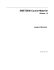### EC327: Advanced Econometrics, Spring 2007 Limited dependent variables and sample selection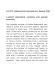### Stata Press presents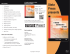### A Multilevel Sample Selection Probit Model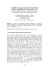### Stata Press presents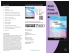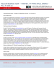### READING EQUINOX FILES INTO STATA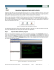### Censored Regression, Sample Selection, Endogenous Switching, and Treatment-Effect Regression Models Dan Powers Soc385K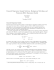### EC327: Financial Econometrics, Spring 2013 Limited dependent variables and sample selection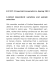### IS THE NFL BETTING MARKET EFFICIENT?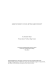### Document 6458595